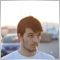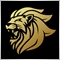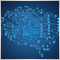# Moving HLine1405

Hi, I wrote this code to mark S/R levels on the chart, but object is not moved at least in backtester:

```   if(Support>0){ // SUPPORT IS OPEN/CLOSE PRICE OF THE LAST CANDLE
ObjectCreate(0,SupportLine,OBJ_HLINE,0,0,Support);
ObjectSet(SupportLine,OBJPROP_COLOR,clrBlue);
if(ObjectMove(0,SupportLine,OBJ_HLINE,0,Support)){ChartRedraw(0);}
}
if(Resistance>0){ // RESISTANCE IS OPEN/CLOSE PRICE OF THE LAST CANDLE
ObjectCreate(0,ResistanceLine,OBJ_HLINE,0,0,Resistance);
ObjectSet(ResistanceLine,OBJPROP_COLOR,clrRed);
if(ObjectMove(0,ResistanceLine,OBJ_HLINE,0,Resistance)){ChartRedraw(0);}
}```

As the iOpen/iClose values change it's not currently moving object. Solution?Moderator
12689

That will generate an error 4200 object already exists watch the log you have to create the object once for example in OnInit and then move it further on in the code, or you have to check it's existence before trying to create it by ObjectFind() function.

Here is a function that moves a Hline.

```//+------------------------------------------------------------------+
//| Move horizontal line                                             |
//+------------------------------------------------------------------+
bool HLineMove(const long   chart_ID=0,   // chart's ID
const string name="HLine", // line name
double       price=0)      // line price
{
//--- if the line price is not set, move it to the current Bid price level
if(!price)
price=SymbolInfoDouble(Symbol(),SYMBOL_BID);
//--- reset the error value
ResetLastError();
//--- move a horizontal line
if(!ObjectMove(chart_ID,name,0,0,price))
{
Print(__FUNCTION__,
": failed to move the horizontal line! Error code = ",GetLastError());
return(false);
}
//--- successful execution
return(true);
}
//+------------------------------------------------------------------+ ```1405

Marco vd Heijden:

That will generate an error 4200 object already exists watch the log you have to create the object once for example in OnInit and then move it further on in the code, or you have to check it's existence before trying to create it by ObjectFind() function.

Here is a function that moves a Hline.

Ok, thank you. But I realized that issue comes from pricing update, that's why line doesn't move.

Use this variables to get the last month's open and closing price but only marks support and resistance level at the moment of backtest is started.

```   double LastOpen=iOpen(Symbol(),PERIOD_MN1,1);
double LastClose=iClose(Symbol(),PERIOD_MN1,1);
string SupportLine="Support",ResistanceLine="Resistance";
bool Bullish=LastClose>LastOpen,Bearish=LastClose<LastOpen;
if(Bullish){Support=LastOpen;Resistance=LastClose;}
if(Bearish){Support=LastClose;Resistance=LastOpen;}```
So may I have to get that values from array?Moderator
12689

There is no array in that code.

The previous code you posted was also incomplete in terms of new value calculation.

Post the relevant code if possible.1405

Marco vd Heijden:

There is no array in that code.

The previous code you posted was also incomplete in terms of new value calculation.

Post the relevant code if possible.

Just have that.Moderator
20375

```//+------------------------------------------------------------------+
//|                                 Horizontal Lines at High Low.mq4 |
//|                                                    Keith Watford |
//+------------------------------------------------------------------+
#property version   "1.00"
#property strict
#property indicator_chart_window
//--- input parameters
input ENUM_TIMEFRAMES      TimeFrame=PERIOD_D1;
input color                HighColour=clrLimeGreen;//High Line Colour
input color                LowColour=clrDodgerBlue;//Low Line Colour
input int                  LineWidth=1;//Line Width

string Prefix="HiLoLine:";
//+------------------------------------------------------------------+
//| Custom indicator initialization function                         |
//+------------------------------------------------------------------+
int OnInit()
{
//--- Draw Horizontal Lines
if(!ObjectCreate(ChartID(),Prefix+"High Line",OBJ_HLINE,0,0,0))
Print(__FUNCTION__,": failed to create the High horizontal line! Error code = ",GetLastError());
else
{
ObjectSetInteger(ChartID(),Prefix+"High Line",OBJPROP_COLOR,HighColour);
ObjectSetInteger(ChartID(),Prefix+"High Line",OBJPROP_WIDTH,LineWidth);
}

if(!ObjectCreate(ChartID(),Prefix+"Low Line",OBJ_HLINE,0,0,0))
Print(__FUNCTION__,": failed to create the Low horizontal line! Error code = ",GetLastError());
else
{
ObjectSetInteger(ChartID(),Prefix+"Low Line",OBJPROP_COLOR,LowColour);
ObjectSetInteger(ChartID(),Prefix+"Low Line",OBJPROP_WIDTH,LineWidth);
}

//---
return(INIT_SUCCEEDED);
}
//+------------------------------------------------------------------+
//| Custom indicator deinitialization function                                 |
//+------------------------------------------------------------------+
void OnDeinit(const int reason)
{
ObjectsDeleteAll(0,Prefix);
//---
}
//+------------------------------------------------------------------+
//| Custom indicator iteration function                              |
//+------------------------------------------------------------------+
int OnCalculate(const int rates_total,
const int prev_calculated,
const datetime &time[],
const double &open[],
const double &high[],
const double &low[],
const double &close[],
const long &tick_volume[],
const long &volume[],
{
static datetime barTime=0;
datetime nowTime=iTime(_Symbol,TimeFrame,1);
if(barTime!=nowTime)
{
barTime=nowTime;
double hi=iHigh(_Symbol,TimeFrame,1);
double lo=iLow(_Symbol,TimeFrame,1);
ObjectSetDouble(ChartID(),Prefix+"High Line",OBJPROP_PRICE,hi);
ObjectSetDouble(ChartID(),Prefix+"Low Line",OBJPROP_PRICE,lo);
}
//--- return value of prev_calculated for next call
return(rates_total);
}
//+------------------------------------------------------------------+
```1405

Keith Watford:

Doesn't update.

```   static datetime barTime=0;
double LastOpen=0,LastClose=0;
datetime nowTime=iTime(Symbol(),Candle,1);
if(barTime!=nowTime){
barTime=nowTime;
LastOpen=iOpen(Symbol(),PERIOD_MN1,1);
LastClose=iClose(Symbol(),PERIOD_MN1,1);
}```Moderator
20375

David Diez:

Doesn't update.

```static datetime barTime=0;
double LastOpen=0,LastClose=0;
datetime nowTime=iTime(Symbol(),Candle,1);
if(barTime!=nowTime){
barTime=nowTime;
LastOpen=iOpen(Symbol(),PERIOD_MN1,1);
LastClose=iClose(Symbol(),PERIOD_MN1,1);
}```

What doesn't update?

Read through the code carefully and understand what it is doing.

Are you actually coding what you want it to do?

Why are you hard-coding the time-frame when you have it in the inputs?

`input ENUM_TIMEFRAMES      TimeFrame=PERIOD_D1;`

Change it to

`input ENUM_TIMEFRAMES      TimeFrame=PERIOD_MN1;`

What is Candle?

`   datetime nowTime=iTime(Symbol(),Candle,1);`1405

Keith Watford:

What doesn't update?

Read through the code carefully and understand what it is doing.

Are you actually coding what you want it to do?

Why are you hard-coding the time-frame when you have it in the inputs?

Change it to

What is Candle?

Candle is timeframe.Moderator
20375

David Diez:
Candle is timeframe.

Why?

The code is very simple and only needs to use 1 time-frame and that is in the inputs.

Run my code untouched through the strategy tester and you will see that it updates the lines every time a new day starts with the previous day's high and low.

Modify the code so that it uses Monthly TF and test, yes, still works.

Then you want to modify it so that it uses Open and Close instead of High and Low.

Test it as you are modifying it and if it stops working correctly ask yourself why.103

```//+------------------------------------------------------------------+
//|
//|
//|
//+------------------------------------------------------------------+

#property link "EA's That Make Money"
#property description "Use on any chart"
#property strict

//--- indicator settings
#property indicator_chart_window
#property indicator_buffers 1
#property indicator_color1  clrNONE
//--- input parameters
extern bool RESETeveryday=false;
extern bool reversebs=false;
extern string lnDescriptionsell="sell-.01";
input string useHLine="LINE2";
input string useHLine2="LINE3";
extern bool useLastHigh=true;
extern bool useLastLow=true;
extern string LoobackPeriod="---- How many bars back to find LLow or HHigh(120/4hour &60 also good)";
extern int LookbackHHigh=60;
extern int LookbackLLow=60;
double last_high=0;
double lastlow=200;

double pt;
double shiftHLine3=0;
int highest1=0,lowest1=0;
double HHigh=0,LLow=0;
datetime period1=0;
datetime period2=0;
//+------------------------------------------------------------------+
//| resetALL
//+------------------------------------------------------------------+
void resetALL()
{
last_high=0;lastlow=200;
highest1=0;lowest1=0;
HHigh=0;LLow=0;
ObjectDelete(useHLine);
ObjectDelete(useHLine2);
}
//+------------------------------------------------------------------+
//| Custom indicator initialization function                         |
//+------------------------------------------------------------------+
void OnInit()
{

if(Digits==3 || Digits==5) pt=10*Point;
else                       pt=Point;

//----
}
//+------------------------------------------------------------------+
//| expert deinitialization function                                 |
//+------------------------------------------------------------------+

int deinit()
{
//----
ObjectDelete(0,useHLine);
ObjectDelete(0,useHLine2);
//----
return(0);
}

int OnCalculate(const int rates_total,
const int prev_calculated,
const datetime &time[],
const double &open[],
const double &high[],
const double &low[],
const double &close[],
const long &tick_volume[],
const long &volume[],
{

highest1=iHighest(NULL,PERIOD_CURRENT,MODE_HIGH,LookbackHHigh,2);
lowest1=iLowest(NULL,PERIOD_CURRENT,MODE_LOW,LookbackLLow,2);
HHigh=High[highest1];
LLow=Low[lowest1];

if(ObjectType(useHLine2)==OBJ_HLINE)lastlow=ObjectGet(useHLine2,OBJPROP_PRICE1);
if(ObjectType(useHLine)==OBJ_HLINE)last_high=ObjectGet(useHLine,OBJPROP_PRICE1);
if(lastlow!=GlobalVariableGet(useHLine2))GlobalVariableSet(useHLine2,lastlow);
if(last_high!=GlobalVariableGet(useHLine))GlobalVariableSet(useHLine,last_high);

if(useLastHigh==true)
{
if(HHigh>last_high)
last_high=HHigh;
}

if(useLastLow==true)
{
if(LLow<lastlow)
lastlow=LLow;
}

if(reversebs==false)
{

ObjectDelete(useHLine2);
ObjectCreate(useHLine2,OBJ_HLINE,0,0,lastlow);
ObjectSet(useHLine2,OBJPROP_COLOR,clrAqua);//clrNONE
ObjectSet(useHLine2,OBJPROP_WIDTH,1);
ObjectSet(useHLine2,OBJPROP_RAY,False);

ObjectDelete(useHLine);
ObjectCreate(useHLine,OBJ_HLINE,0,0,last_high);
ObjectSetText(useHLine,lnDescriptionsell);
ObjectSet(useHLine,OBJPROP_COLOR,clrYellow);
ObjectSet(useHLine,OBJPROP_WIDTH,1);
ObjectSet(useHLine,OBJPROP_RAY,False);
}
if(reversebs==true)
{

ObjectDelete(useHLine2);
ObjectCreate(useHLine2,OBJ_HLINE,0,0,lastlow);
ObjectSetText(useHLine2,lnDescriptionsell);
ObjectSet(useHLine2,OBJPROP_COLOR,clrAqua);//clrNONE
ObjectSet(useHLine2,OBJPROP_WIDTH,1);
ObjectSet(useHLine2,OBJPROP_RAY,False);

ObjectDelete(useHLine);
ObjectCreate(useHLine,OBJ_HLINE,0,0,last_high);
ObjectSet(useHLine,OBJPROP_COLOR,clrYellow);
ObjectSet(useHLine,OBJPROP_WIDTH,1);
ObjectSet(useHLine,OBJPROP_RAY,False);
}

period1=iTime(NULL,PERIOD_D1,1);
if(period1>period2 && RESETeveryday==true)
{
resetALL();
period2=period1;

}

return(rates_total);
}

//+------------------------------------------------------------------+
```
Not sure if this will help. Very simple code. Moves the line and can update every day.